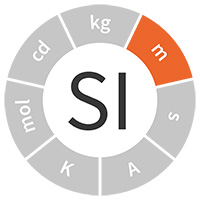Close
Close
SI units

# metre (m)

The metre is the SI unit of lengthWe measure distances by comparing objects or distances with standard lengths. Historically, we used pieces of metal or the wavelength of light from standard lamps as standard lengths. Since the speed of light in vacuum, c, is constant, in the SI we use the distance that light travels in a given time as our standard.

For long distances, such as from the Earth to the moon, we simply measure the time light takes to travel the distance using an accurate timer; for shorter distances (when the time is too short to measure accurately enough), we use laser-based systems, where we ‘count’ the number of wavelengths of the laser light that correspond to the length we want to measure.

## Definition

The metre is defined by taking the fixed numerical value of the speed of light in vacuum, 𝒸, to be 299 792 458 when expressed in the unit m s−1, where the second is defined in terms of the caesium frequency ∆ν.

The wording of the definition was updated in 2019.

Application

We can traceably measure distances from a few picometres (1 × 10–12 m, one trillionth of a metre) up to the distance of the Voyager 1 spacecraft from Earth (already over 2.1 × 1013 m away).

The Large Hadron Collider particle accelerator in Switzerland is installed in 30 km of underground tunnels. To make it work, the steering magnets must be positioned to an accuracy of one tenth of a millimetre every 100 m.

## Did you know?

• The metre was initially defined as one ten-millionth of the distance on the Earth's surface from the north pole to the equator, on a line passing through Paris. Expeditions from 1792 to 1799 determined this length by measuring the distance from Dunkirk to Barcelona, with an accuracy of about 0.02%
• In 1848–49, physicist Hippolyte Fizeau measured the speed of light using a rotating cogwheel. In 2009, NPL and the BBC used a kitchen blender to recreate Fizeau’s experiment

The science behind the unit

Since 1983, the metre has been internationally defined as the length of the path travelled by light in vacuum during a time interval of 1/299 792 458 of a second. This definition can be realised simply and accurately using modern techniques and the speed of light is regarded to be a universal constant, making it ideal as the basis for a length standard.

The definition of the metre can be practically realised in two different ways:

Time of flight – a pulse of light is sent over the length to be measured. The time it takes for the light to travel this distance, in seconds, multiplied by the speed of light (299 792 458 metres per second), gives the length in metres. As the speed of light is very fast, this method is easier to apply over long distances. However, care has to be taken to account for gravitational field effects when measuring astronomical distances.

Interferometry – the technique of interferometry allows a length to be measured in terms of the wavelength of light. By using a light source of known and stable wavelength, lengths up to 100 metres can be directly measured, with accuracies up to 1 part in a few million.

Accurate length measurement and precise definition are needed throughout the modern world. From the tiniest features on a microchip, through standard threads on nuts and bolts, to large, complex sub-assemblies of modern airliners, interchangeability and reproducibility are essential in a global economy where items are sourced from different countries, yet have to fit together perfectly, first time.

Lasers currently used to realise the metre provide very stable optical frequencies (or vacuum wavelengths) by servo-controlling the light that they emit to particular reference absorptions in gases such as iodine, held within small gas cells. However, their accuracies are limited by the motion of the gas molecules in the laser beam at room temperature. By replacing iodine molecules with atoms or ions such as ytterbium or strontium that are held within electromagnetic or optical traps, it is possible to 'laser cool' atoms close to absolute zero (thereby reducing their motion). In this arrangement the optical reference frequencies can be several orders of magnitude more accurate, but other limitations, such as atmospheric conditions or material stability, generally prevent their use in direct length measurement at these improved levels.

Find out about NPL's research in this area